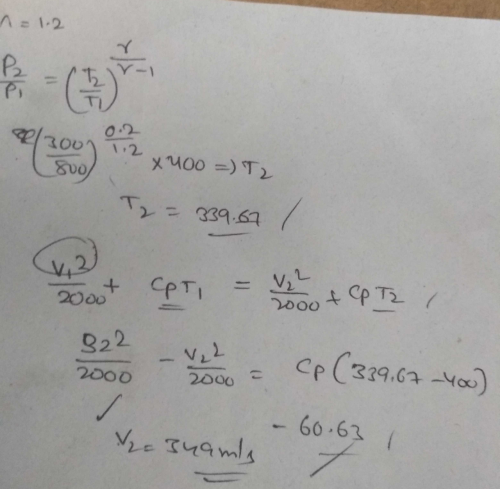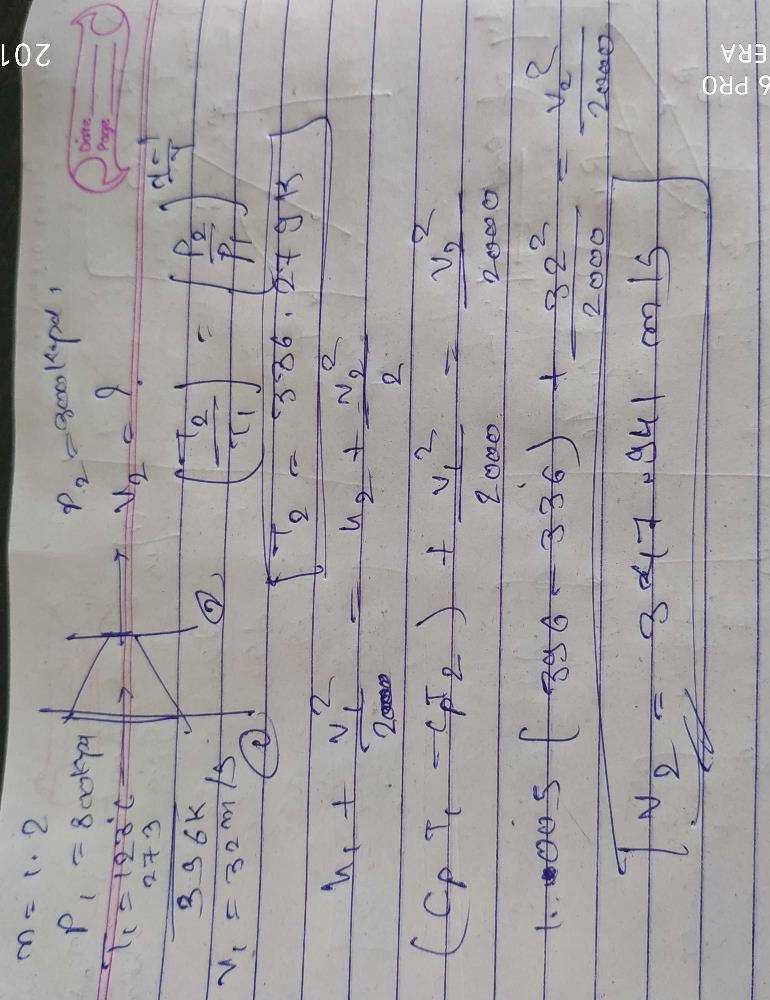Courses

# Air undergoes a polytropic process in an adiabatic nozzle with n = 1.2. Inlet state of air is 800 kPa, 1230C with a velocity of 32 m/s and exit state is 300 kPa. Find out the velocity of air at nozzle exit.a)654 m/sb)348 m/sc)244 m/sd)436 m/sCorrect answer is 'B'. Can you explain this answer? Related Test: Basic Thermodynamics

## Mechanical Engineering Question

Achuth Kumar Yelleti answered Sep 20, 2018Sabir Hussain answered Jul 20, 2018
From P, T, n relationship, we can find T2. So dh can be found out using CpdT. Using dh value in SFEE exit velocity will be found out as 347.94 m/s

Vivek Sharma answered Jun 12, 2018
Just equate : V2^2 + V1^2 = 2×Cp×(T1-T2) and T2 you will get from adiabatic equation as 340 K

Bhupesh Sonkar answered Sep 05, 2019Shyodanaram Baror answered Sep 26, 2020
348

Rapuru Bhavanisankar answered Aug 20, 2020
Exit velocity of air=348m/s
proof: For polytropic process
T2/T1 ={p2/p1}^(n-1/n)
T2=
here n=1.2 (polytropic index) given,
p1 and p2 are also given which are initial and final pressure respectively,
initial temperature t1=123 C
T1=123+273=396K
from above formula we get final temperature T2=336.28K
h1-h2=((v2^2)/2000)-((v1^2)/2000)
here v is velocity
h1-h2=mCp∆T=60.02kJ
Cp=1.005 for air
By above formulae
velocity V2=347.94m/s